﻿ Artificial neural network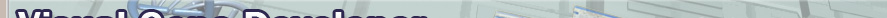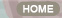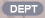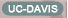Home > Features > 9.Artificial neural network

The artificial neural network prediction tool

For data regression and prediction, Visual Gene Developer includes an artificial neural network toolbox. You can easily load data sets to spreadsheet windows and then correlate input parameters to output variables (=regression or learning) on the main configuration window. Because the software provides a specialized class whose name is 'NeuralNet', users can directly access to the class to make use of neural network prediction toolbox when they develop new modules. A user can use maximum 5 instances of NeuralNet including 'NeuralNet', 'NeuralNet2', 'NeuralNet3', 'NeuralNet4', and 'NeuralNet5'.

We used a typical feed-forward neural network with a standard backpropagation learning algorithm to train networks and provides several different transfer functions. Without using gene design or optimization, our neural network package works perfectly independently even though all menus are still in the software environment. In this section, we shortly describe the artificial neural networks and then demonstrate how to use neural network toolbox and the class.

New update: if you are a programmer and want to use trained neural network files in your own programs, check NeuralNet.java.

Visual Gene Developer is a free software for artificial neural network prediction for general purposes!!!

Check built-in analysis tools: data normalization, pattern analysis, network map analysis, regression analysis, programming function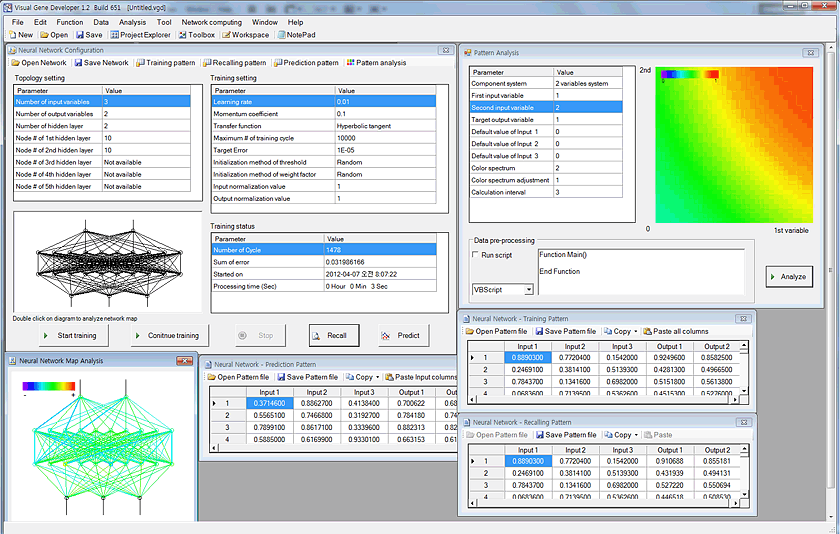o Artificial neural network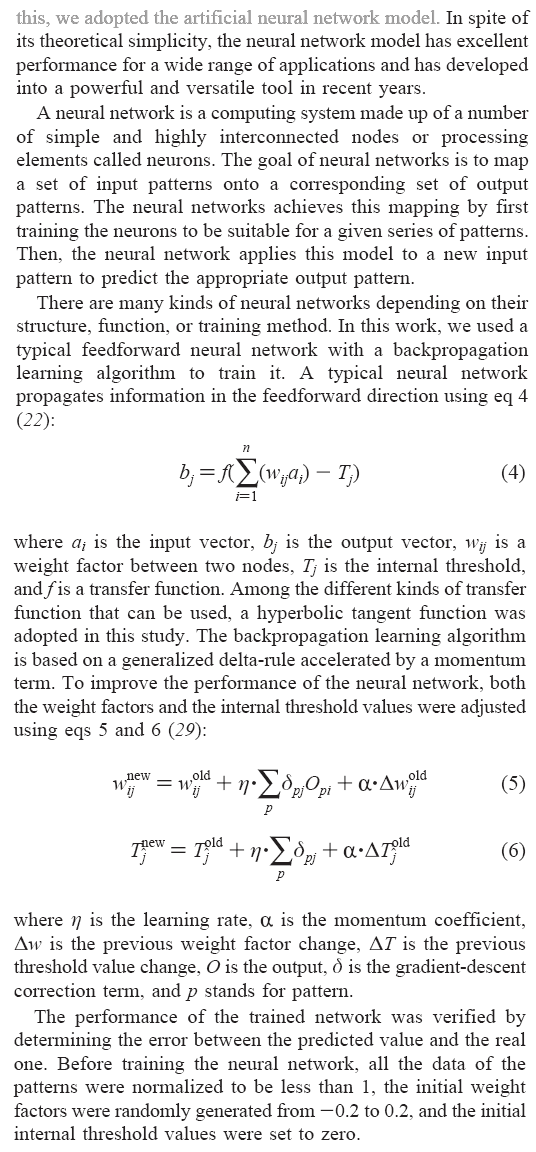From Sang-Kyu Jung & Sun Bok Lee, Biotechnology Progress, 2006.

Simple slides here.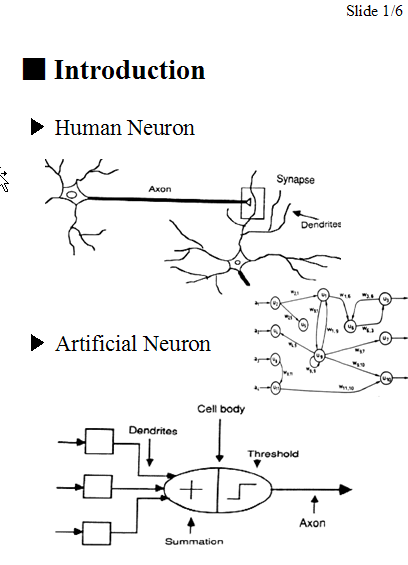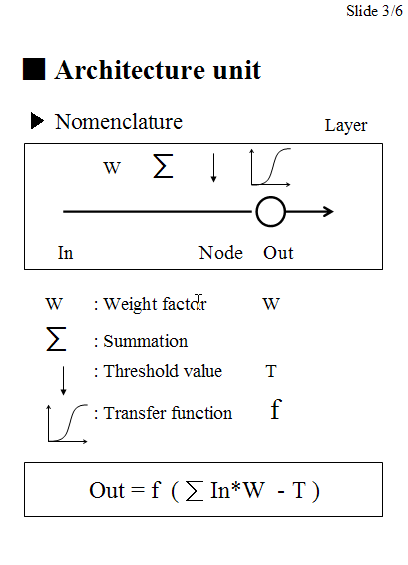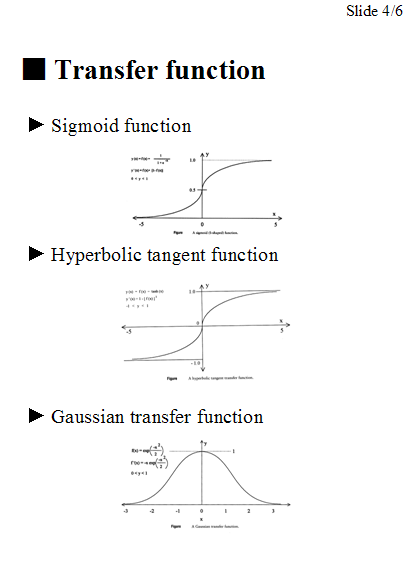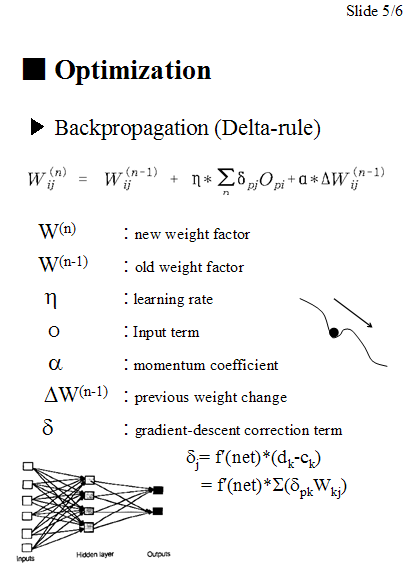o How to use artificial neural network toolbox

Step 1: Prepare data set

In the 'Equation', 'Calculated Output1' and 'Calculated Output2' were divided by 2 or 3 to normalize data. Keep in mind that all data values should be less than 1 and must be normalized if they are bigger than 1. If the numbers are higher than 1 it may mean that they are out of range for the neural network prediction.

New update!     A new function for data normalization has been implemented!

 Equation Input1=Rand()   'random number between 0 and 1  Input2=Rand()   'random number between 0 and 1  Input3=Rand()   'random number between 0 and 1  Calculated Output1=(Input1+Input2^Input3)/2  Calculated Output2=(Input1+Sin(Input2)+Cos(Input3))/3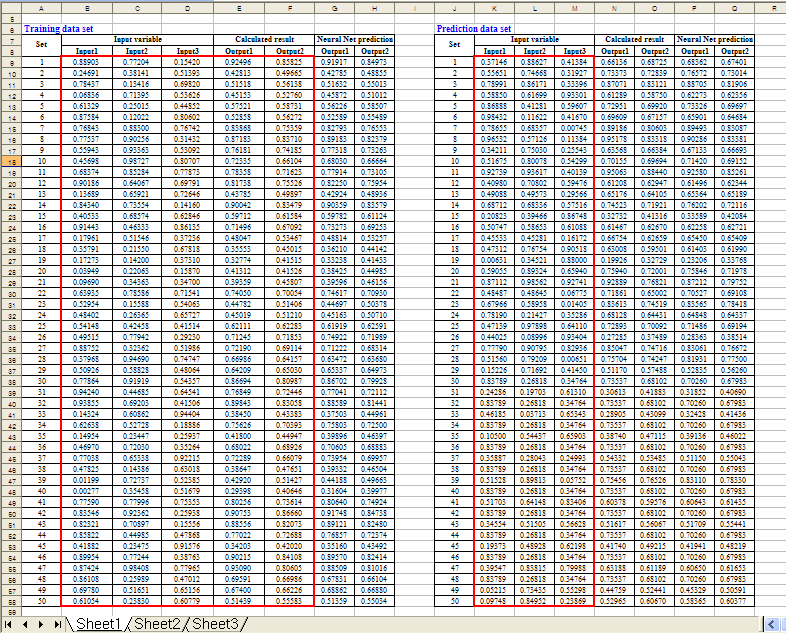Step 2: Configure a neural network

1. Click the 'Artificial neural network' in the 'Tool' menu

2. You can see the window titled 'Neural Network Configuration'. Adjust parameters as shown in the 'Topology setting' and 'Training setting'

3. First, click on the 'Training pattern' button in order to set up the training data set. Immediately, you can see a new pop-up window. But it doesn't include any data initially.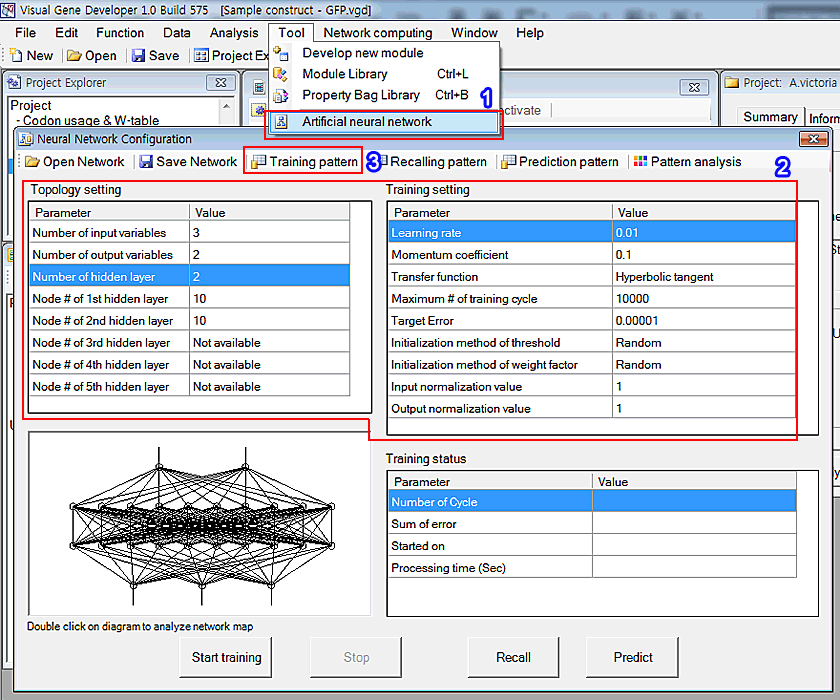The sum of error is defined by the following equation.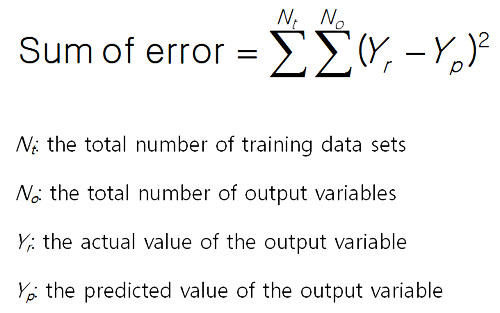4. Copy the following region of the training data set in the Excel document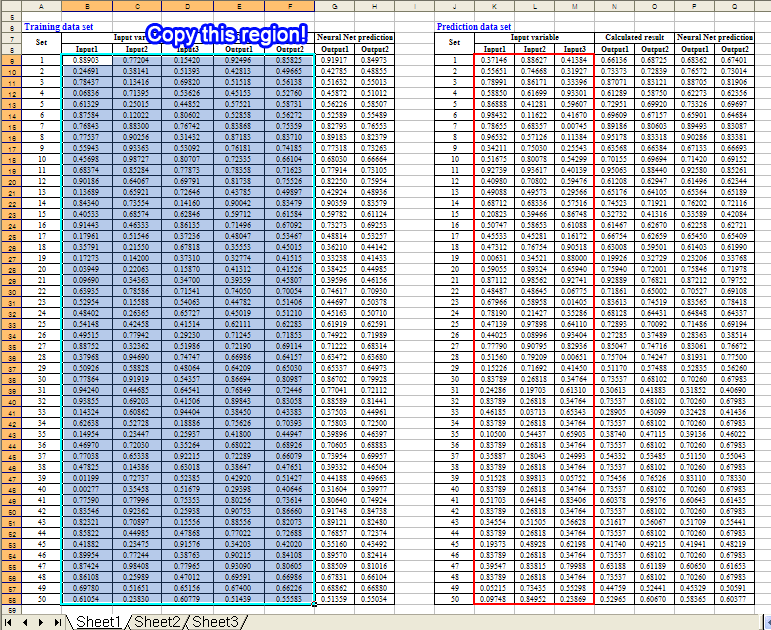5. Click on the 'Paste all columns' button in the 'Neural Network - Training Pattern' window. It retrieves text data from the clipboard and pastes it to the table as shown in the figure.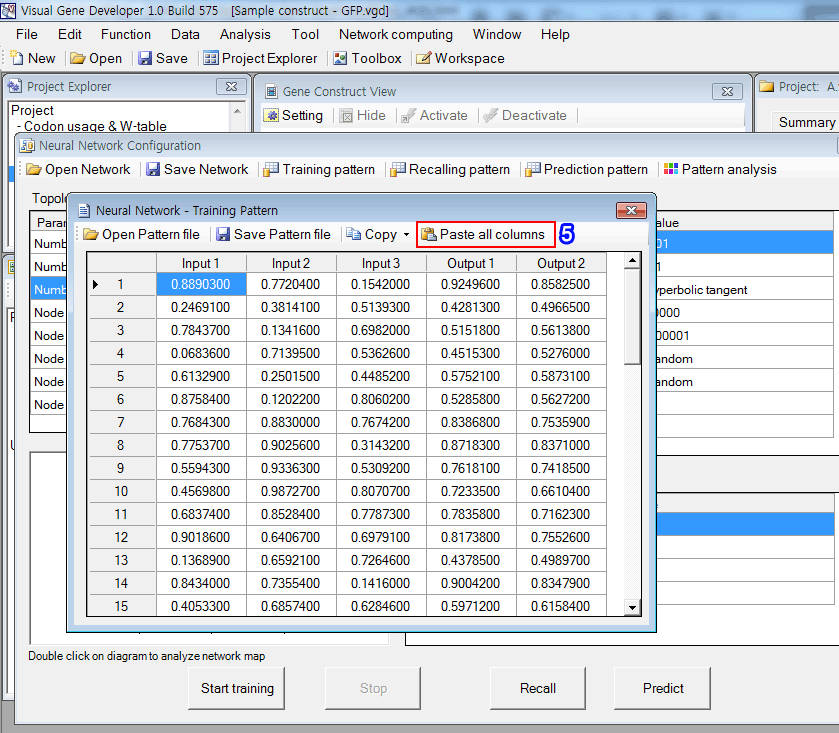Step 3: Start learning process (=data regression)

1. Click on the 'Start training' button. It took about 70 seconds to repeats 30,000 cycles.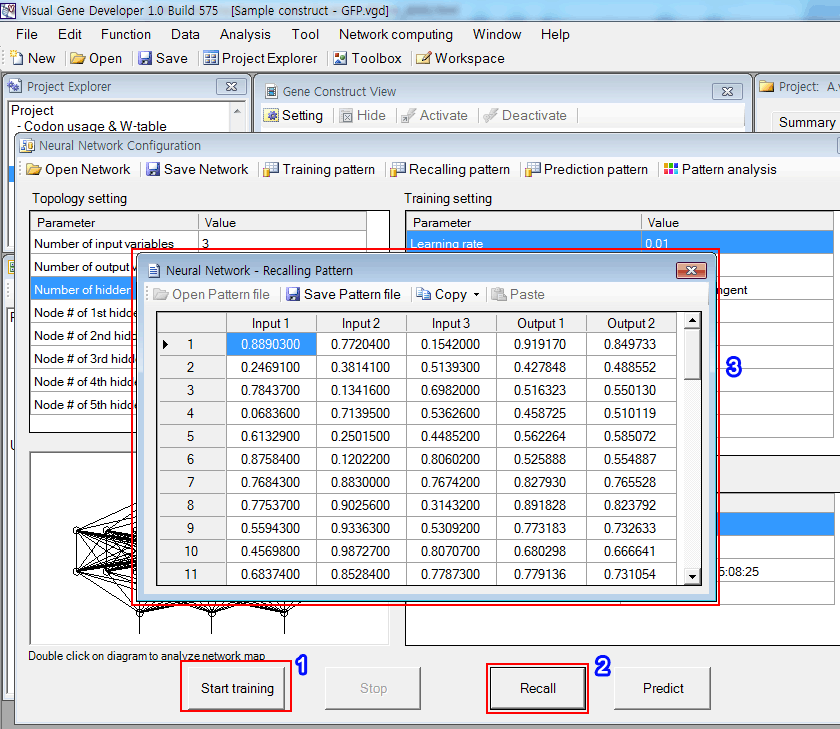2. Click on the 'Recall' button.

3. The software filled the empty columns (Outpu1 and Output2) with numbers and you can check the predicted values. The 'Copy' button is available.

4. The regression result is shown in the below figure. It looks quite good.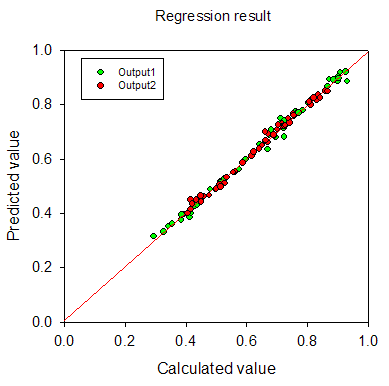Step 4: Predict new data set

1. Copy the following region of the training data set in the Excel document.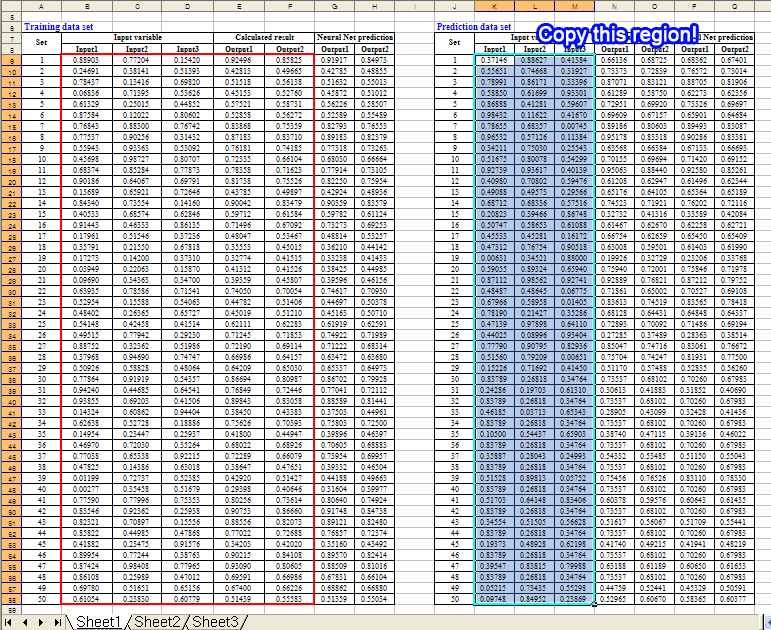2. Click on the 'Prediction pattern' button in the 'Neural Network Configuration' window

3. Click on the 'Paste Input columns' button to paste data of clipboard to the table

4. Click on the 'Predict' button. It will complete the table as shown in the figure. You can check the predicted values.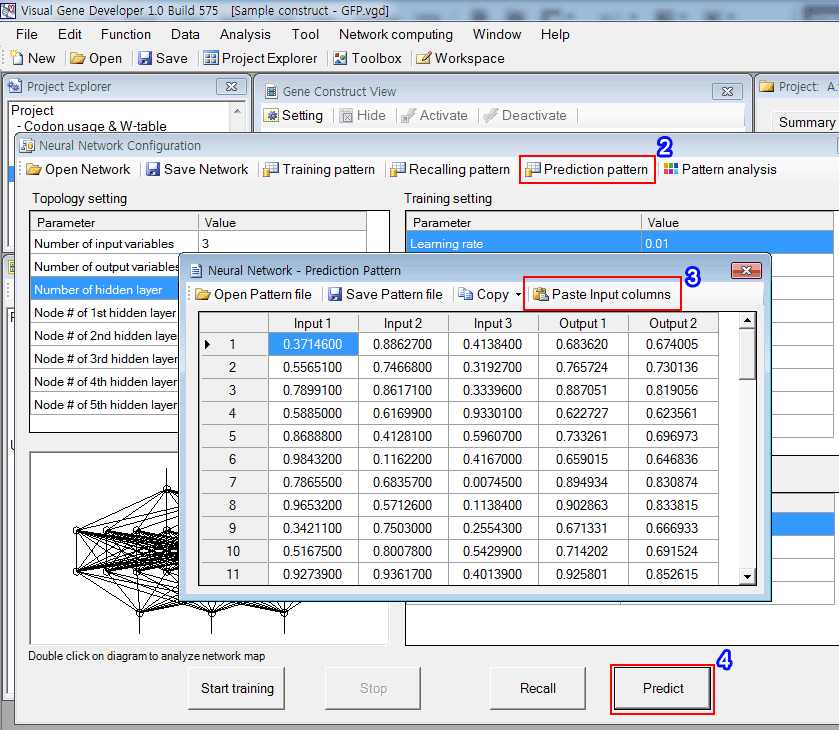5. The result is shown in the figure. It really works well.o Data normalization

- Click on the 'Normalize' button to show the pop-up window.o Pattern analysis

In the case of multiple input variable systems, Visual Gene Developer provides a useful function to test 2 or 3 input variables as a nice plot.

2-D plot for two-variable system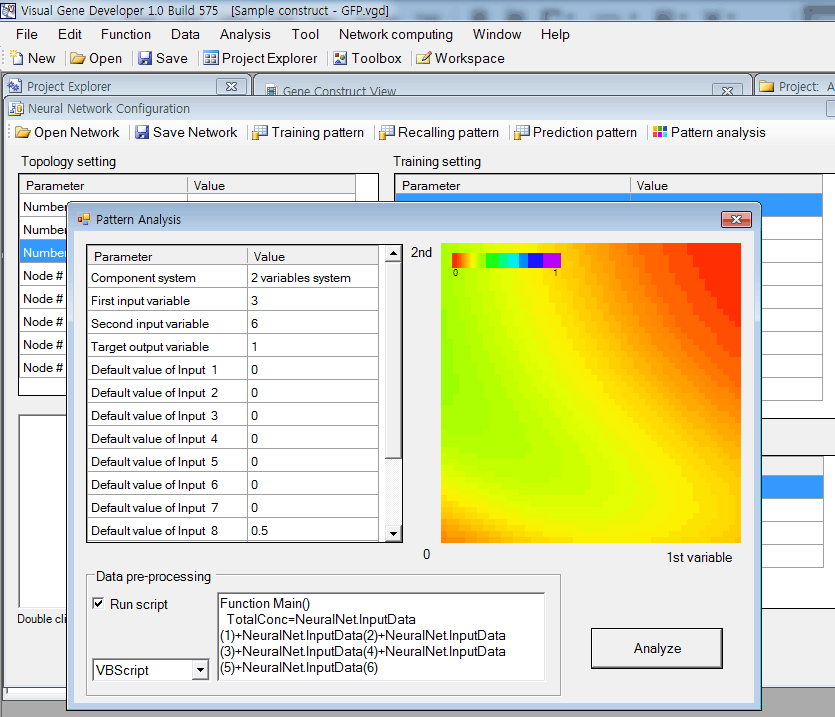Ternary plot for three input variable system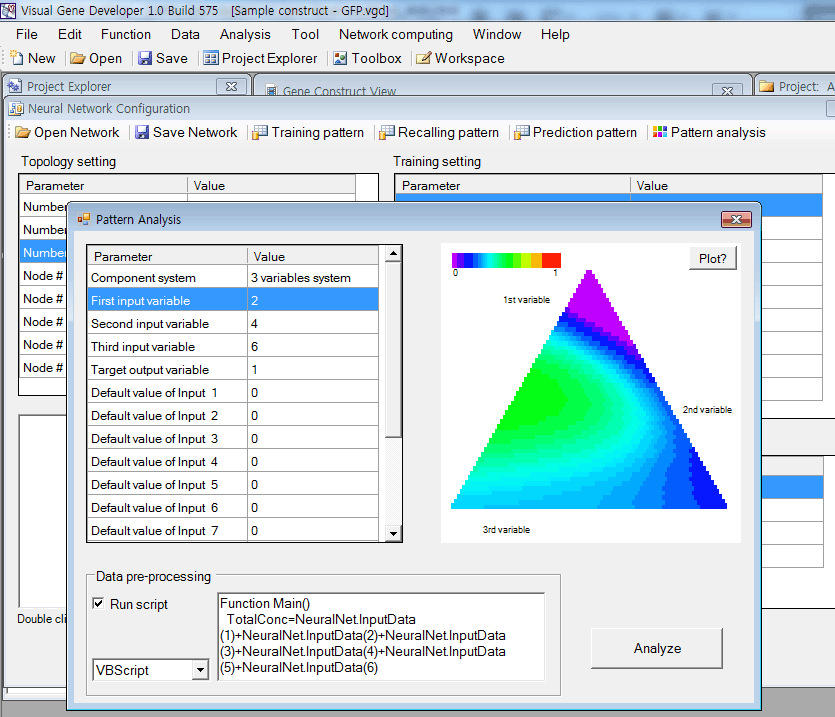'Data pre-processing' is performed if 'Run script' is checked.

Internally, Visual Gene Developer assigns initial values of all input variables and then executes the script code written in 'Data pre-processing'.

This function is useful when a certain input variable depends on other variables. For example, input 3 is the sum of input 1 and input 2.

To adjust the value of input 3, you can write code like,

 Function Main()    NeuralNet.InputData(3)=NeuralNet.InputData(1)+NeuralNet.InputData(2) End Function

Visual Gene Developer provides a graphical visualization of a trained network for a user. You can check the color and width of a line or circle.

Lines represent weight factors and circles (node) mean threshold values.Just double-click on a diagram in the 'Neural Network Configuration' window.

In the diagram, the red color corresponds to a high positive number and violet color means a high negative number. Line width is proportional to the absolute number of  weight factor or threshold value.

o Regression analysis   New update!You can save the data set table as a standard comma delimited text file. Our neural network (trained) data file is also easily accessible because it has a standard text file format. You can open sample files and check the content.

Although Visual Gene Developer has a user-friendly neural network toolbox, a user may prefer using the 'NeuralNet' class to make customized analysis module. A user can use maximum 5 instances of NeuralNet including 'NeuralNet', 'NeuralNet2', 'NeuralNet3', 'NeuralNet4', and 'NeuralNet5'.

Example

1. Click on the 'Module Library' in the 'Tool' menu

2. Choose the 'Sample NeuralNet' item in the 'Module Library' window

3. Click on the 'Edit Module' button in the 'Module Library' window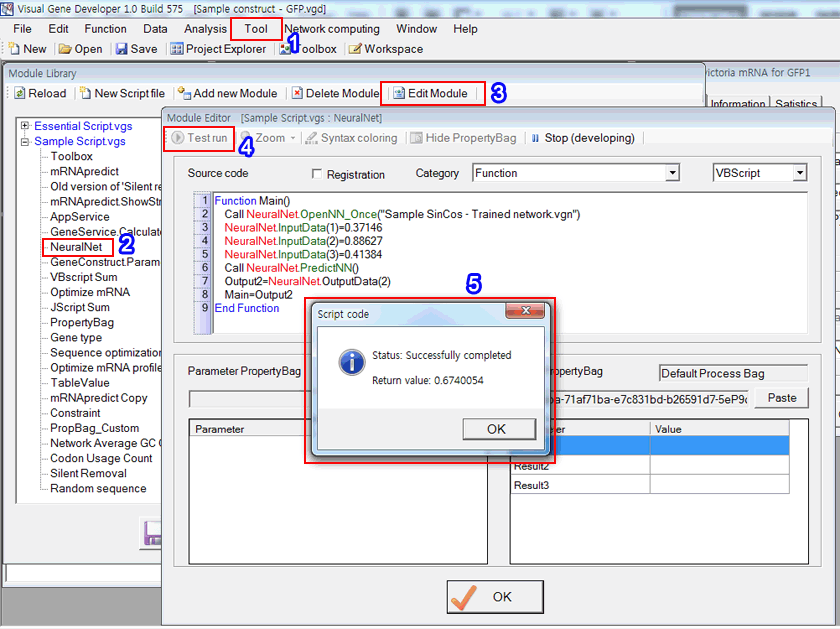4. Click on the 'Test run' button in the 'Module Editor' window.  Check source code and explanation!

Source code

VBScript

 Function Main()   Call NeuralNet.OpenNN_Once("Sample SinCos - Trained network.vgn")      '<---  Open trained neural network file   NeuralNet.InputData(1)=0.37146       '<---  Set value to Input1  (it's the first data set in prediction date table)   NeuralNet.InputData(2)=0.88627       '<---  Set value to Input2  (it's the first data set in prediction date table)   NeuralNet.InputData(3)=0.41384       '<---  Set value to Input3  (it's the first data set in prediction date table)   Call NeuralNet.PredictNN()                '<---  Predict data   Output2=NeuralNet.OutputData(2)     '<---  Get value of Output2   Main=Output2                                     '<---  Return Output2 value End Function

JScript

 function Main() {   m=NeuralNet.OpenNN_Once ( "Sample SinCos - Trained network.vgn" );       //<--- Open trained neural network file   NeuralNet.InputData(1)=0.37146;       //<--- Set value to Input1 (it's the first data set in prediction date table)   NeuralNet.InputData(2)=0.88627;       //<--- Set value to Input2 (it's the first data set in prediction date table)   NeuralNet.InputData(3)=0.41384;       //<--- Set value to Input3 (it's the first data set in prediction date table)   NeuralNet.PredictNN;                         //<--- Predict data   Output2=NeuralNet.OutputData(2);     //<--- Get value of Output2   return Output2;                                     //<--- Return Output2 value }

5. The 'Return message' shows a result.  It's the same value as shown in the previous prediction date table.